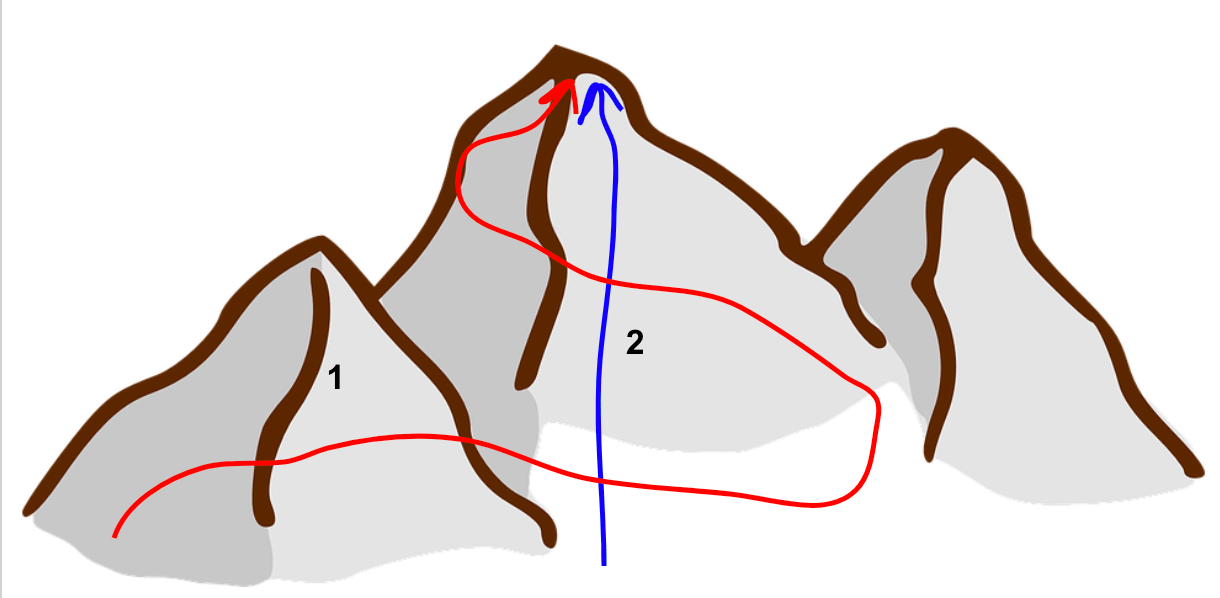Cyber Sale Extended 3 Days: ALL MCAT Products & Admissions Services

MCAT Content / Energy Changes In Chemical Reactions / Thermodynamic System State Functions

### Thermodynamic System: State Functions

Topic: Energy Changes In Chemical Reactions

In thermodynamics, a state function is a property that is defined only by the current state of the system rather than the changes that occurred between the initial and final state; Measurements that can be defined as state functions are density, volume, temperature, and pressure.

The state function of a system is defined as the difference between the initial and final value of a function (e.g. Tfinal – Tinitial). An example of this would be thinking about two different paths to the top of a mountain, 1 and 2:Even though path 1 is longer and more winded than path 2, both paths lead to the same destination. In this case, although the two paths are different, their final change in altitude is the same. Hence, they have the same state function value for altitude.

In a lab setting, if the volume of container A has an initial volume of 1L and immediately expands to 2L, the net change is 1L. In container B, if the volume has an initial value of 1L and fluctuates from 1L to 5L, 5L to .5L, and .5L to 2L, the final volume is 2L, and the net change is 1L. Containers A and B differed in their volume change paths, but because the initial and final volumes of the containers are the same, their net change is the same, hence affirming volume as a state function along with the other measurements listed above.

Some examples of common state functions are ΔH (enthalpy), ΔS (entropy), ΔG (free energy change). All of these values can be measured in thermodynamic calculations using their initial and final values.

Key Points

• State functions only consider the initial and final state of a system, not the states in between; in other words, they only consider current states.

• Density, volume, temperature, and pressure are state functions

Key Terms

state function: property that depends only on the initial and final state of a system

Billing Information